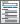Home  /  Products  /  Features  /  Generalized linear models

## Generalized linear models

• Identity
• Log
• Logit
• Probit
• Complementary log-log
• Power
• Odds power
• Negative binomial
• Log-log
• Log-complement
• Gaussian (normal)
• Inverse Gaussian
• Bernoulli/binomial
• Poisson
• Negative binomial
• Gamma

Choice of estimation method

Customizable functions• User-defined variance functions
• User-defined HAC kernels

Choice of variance estimates and standard errors• Inverse Hessian
• Outer product of the gradients (OPG)
• Observed information matrix
• Expected information matrix
• Robust Huber/White/sandwich estimator
• Robust variance with clustered/correlated data
• Heteroskedasticity- and autocorrelation-consistent (HAC) with Newey–West, Gallant, Anderson, or community-contributed kernel
• Jackknife
• Bootstrap
• Correlation structures
• Exchangeable
• Independent
• Unstructured
• Autoregressive
• Stationary
• Nonstationary
• Fixed
• Conventional, robust, bootstrap, and jackknife standard errors

Multilevel mixed-effects GLMs

• View and run all postestimation features for your command
• Automatically updated as estimation commands are run
• Expected value of dependent variable
• Anscombe residual
• Cook’s distance
• Deviance residual
• Diagonal of hat matrix
• Likelihood residual
• Pearson residual
• Response residual
• Score residual
• Working residual
• Automatically create indicators based on categorical variables
• Form interactions among discrete and continuous variables
• Include polynomial terms
• Perform contrasts of categories/levels
Watch Introduction to Factor Variables in Stata tutorials
Watch Introduction to margins in Stata tutorials
Watch Profile plots and interaction plots in Stata tutorials